SNVS549C June   2009  – February 2016

PRODUCTION DATA.

1. Features
2. Applications
3. Description
4. Revision History
5. Specifications
6. Detailed Description
1. 6.1 Overview
2. 6.2 Functional Block Diagram
3. 6.3 Feature Description
4. 6.4 Device Functional Modes
5. 6.5 Programming
1. 6.5.1 I2C-Compatible Interface
6. 6.6 Register Maps
1. 6.6.1 Register Descriptions
7. Application and Implementation
1. 7.1 Application Information
2. 7.2 Typical Application
1. 7.2.1 Design Requirements
2. 7.2.2 Detailed Design Procedure
3. 7.2.3 Application Curves
8. Power Supply Recommendations
9. Layout
10. 10Device and Documentation Support
1. 10.1 Device Support
2. 10.2 Documentation Support
3. 10.3 Community Resources
5. 10.5 Electrostatic Discharge Caution
6. 10.6 Glossary
11. 11Mechanical, Packaging, and Orderable Information

• YFQ|16

## 7 Application and Implementation

NOTE

Information in the following applications sections is not part of the TI component specification, and TI does not warrant its accuracy or completeness. TI’s customers are responsible for determining suitability of components for their purposes. Customers should validate and test their design implementation to confirm system functionality.

### 7.1 Application Information

The LM3554 is a dual-string white-LED driver for LED camera flash applications. The dual high-side current sources allow for grounded cathode LEDs. The integrated boost provides the power for the current sources and can source up to 1.2 A from a single-cell Li+ voltage range.

### 7.2 Typical Application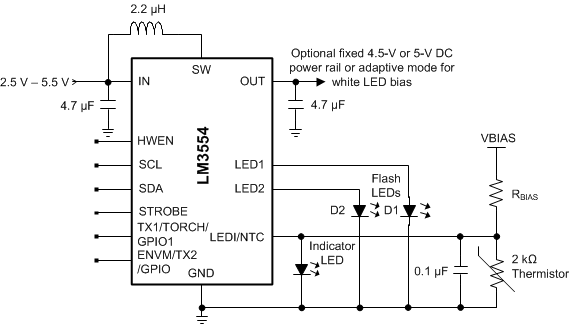Figure 41. LM3554 Typical Application

#### 7.2.1 Design Requirements

For typical LM3554 device applications, use the parameters listed in Table 10.

### Table 10. Design Parameters

DESIGN PARAMETER EXAMPLE VALUE
Minimum input voltage 2.5 V
Programmable output voltage 4.5 V or 5 V
Programmable output current 37.5 mA to 1.2 A

### Table 11. Application Circuit Component List

COMPONENT MANUFACTURER VALUE PART NUMBER SIZE (mm) RATING
L TOKO 2.2 µH FDSE0312-2R2M 3 × 3 × 1.2 2.3 A (0.2 Ω)
COUT Murata 4.7 µF/10 µF GRM188R60J475M, or GRM188R60J106M 0603 (1.6 × 0.8 ×0.8 ) 6.3 V
CIN Murata 4.7 µF GRM185R60J475M 0603 (1.6 × 0.8 × 0.8 ) 6.3 V
LEDs Lumiled LXCL-PWF4 1.5 A

#### 7.2.2.1 Output Capacitor Selection

The LM3554 is designed to operate with a at least a 4.7-µF ceramic output capacitor in LED mode and a 10-µF output capacitor in voltage-output mode. When the boost converter is running the output capacitor supplies the load current during the boost converters on-time. When the NMOS switch turns off the inductor energy is discharged through the internal PMOS switch supplying power to the load and restoring charge to the output capacitor. This causes a sag in the output voltage during the on time and a rise in the output voltage during the off time. The output capacitor is therefore chosen to limit the output ripple to an acceptable level depending on load current and input/output voltage differentials and also to ensure the converter remains stable.

For proper LED operation the output capacitor must be at least a 4.7-µF ceramic (10-µF in voltage-output mode). Larger capacitors such as 10 µF or 22 µF can be used if lower output voltage ripple is desired. To estimate the output voltage ripple considering the ripple due to capacitor discharge (ΔVQ) and the ripple due to equivalent series resistance (ESR) of the capacitor (ΔVESR) use Equation 1 and Equation 2:

For continuous conduction mode, the output voltage ripple due to the capacitor discharge is:

Equation 1.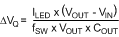The output voltage ripple due to the output capacitors ESR is found by:

Equation 2.In ceramic capacitors the ESR is very low, thus the assumption is that that 80% of the output voltage ripple is due to capacitor discharge and 20% from ESR. Table 12 lists different manufacturers for various output capacitors and their case sizes suitable for use with the LM3554.

#### 7.2.2.2 Input Capacitor Selection

Choosing the correct size and type of input capacitor helps minimize the voltage ripple caused by the device boost converter switching and reduces noise on the devices input terminal that can feed through and disrupt internal analog signals. In the Figure 41 a 4.7-µF ceramic input capacitor works well. It is important to place the input capacitor as close to the device input (IN) terminals as possible. This reduces the series resistance and inductance that can inject noise into the device due to the input switching currents. Table 12 lists various input capacitors that or recommended for use with the LM3554.

### Table 12. Recommended Input/Output Capacitors (X5R Dielectric)

MANUFACTURER PART NUMBER VALUE CASE SIZE (mm) VOLTAGE RATING
TDK Corporation C1608JB0J475K 4.7 µF 0603 (1.6 × 0.8 × 0.8 ) 6.3 V
TDK Corporation C1608JB0J106M 10 µF 0603 (1.6 × 0.8 × 0.8 ) 6.3 V
TDK Corporation C2012JB1C475K 4.7 µF 0805 (2 ×1.25 ×1.25) 16 V
TDK Corporation C2012JB1A106M 10 µF 0805 (2 ×1.25 ×1.25) 10 V
TDK Corporation C2012JB0J226M 22 µF 0805 (2 ×1.25 ×1.25) 6.3 V
Murata GRM188R60J475KE19 4.7 µF 0603 (1.6 × 0.8 × 0.8 ) 6.3 V
Murata GRM21BR61C475KA88 4.7 µF 0805 (2 ×1.25 ×1.25) 16 V
Murata GRM21BR61A106KE19 10 µF 0805 (2 ×1.25 ×1.25) 10 V
Murata GRM21BR60J226ME39L 22 µF 0805 (2 ×1.25 ×1.25) 6.3 V

#### 7.2.2.3 Inductor Selection

The LM3554 is designed to use a 2.2-µH inductor. Table 13 lists various inductors and their manufacturers that can work well with the LM3554. When the device is boosting (VOUT > VIN) the inductor is typically the biggest area of efficiency loss in the circuit. Therefore, choosing an inductor with the lowest possible series resistance is important. Additionally, the saturation rating of the inductor must be greater than the maximum operating peak current of the LM3554. This prevents excess efficiency loss that can occur with inductors that operate in saturation and prevents over heating of the inductor and possible damage. For proper inductor operation and circuit performance ensure that the inductor saturation and the peak current limit setting of the LM3554 is greater than IPEAK can be calculated by:

Equation 3.where

### Table 13. Recommended Inductors

MANUFACTURER L PART NUMBER DIMENSIONS (L×W×H)(mm) ISAT
TOKO 2.2 µH FDSE0312-2R2M 3 × 3 ×1.2 2 A
TDK 2.2 µH VLS252012T-2R2M1R3 2 × 2.5 ×1.2 mm 1.5 A
Coilcraft 2. 2µH LPS4018-222ML 3.9 × 3.9 × 1.7 mm 2.3 A

#### 7.2.2.4 NTC Thermistor Selection

NTC thermistors have a temperature to resistance relationship of:

Equation 4.where

• β is given in the thermistor datasheet
• R25C is the thermistors value at 25°C

Figure 43 is chosen so that it is equal to:

Equation 5.where

• R(T)TRIP is the thermistor value at the temperature trip point
• VBIAS is shown in Figure 43
• VTRIP = 1.05V (typical)

Choosing R3 here gives a more linear response around the temperature trip voltage. For example, with VBIAS = 2.5 V, a thermistor whose nominal value at 25°C is 100 kΩ and a β = 4500 K, the trip point is chosen to be 93°C. The value of R(T) at 93°C is:

Equation 6.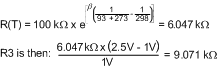Figure 42 shows the linearity of the thermistor resistive divider of the previous example.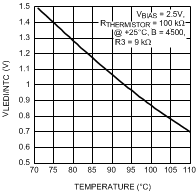Figure 42. Thermistor Resistive Divider Response vs Temperature

Another useful equation for the thermistor resistive divider is developed by combining the equations for R3, and R(T) and solving for temperature. This is shown in Equation 7:

Equation 7.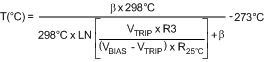Using, for example, Excel® spreadsheet software, different curves for the temperature trip point T (°C) can be created vs R3, Beta, or VBIAS in order to help better choose the thermal components for practical values of thermistors, series resistors (R3), or reference voltages VBIAS.

Programming bit  of the Configuration Register with a 1 selects thermal comparator mode making the LEDI/NTC pin a comparator input for flash LED thermal sensing. Figure 43 shows the internal block diagram of the thermal sensing circuit which is OR’d with both the TX1 and ENVM/TX2 (TX2 mode) to force the LM3554 from flash to torch mode. This is intended to prevent LED overheating during flash pulses.Figure 43. Thermistor Voltage Divider and Sensing Circuit

#### 7.2.2.5 NTC Thermistor Placement

The termination of the thermistor must be done directly to the cathode of the flash LED in order to adequately couple the heat from the LED into the thermistor. Consequently, the noisy environment generated from the boost converter switching can introduce noise from GND into the thermistor sensing input. To filter out this noise it is necessary to place a 0.1-µF or larger ceramic capacitor close to the LEDI/NTC pin. The filter capacitor's return must also connect with a low-impedance trace, as close to the PGND pin of the device as possible.

#### 7.2.2.6 Maximum Load Current (Voltage Mode)

Assuming the power dissipation in the LM3554 and the ambient temperature are such that the device does not hit thermal shutdown, the maximum load current as a function of IPEAK is:

Equation 8.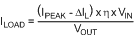where

and

Equation 9.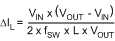Figure 44 shows the theoretical maximum output current vs theoretical efficiency at different input and output voltages using Equation 8 and Equation 9 for ΔIL and ILOAD with a peak current of 2.5 A. Figure 44 represents the theoretical maximum output current (for the LM3554 in voltage-output mode) that the device can deliver just before hitting current limit.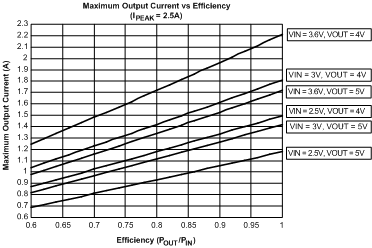Figure 44. LM3554 Maximum Output Current

#### 7.2.2.7 Maximum Output Power

Output power is limited by three things: the peak current limit, the ambient temperature, and the maximum power dissipation in the package. If the LM3554’s die temperature is below the absolute maximum rating of 125°C, the maximum output power can be over 6 W. However, any appreciable output current causes the internal power dissipation to increase and therefore increase the die temperature. This can be additionally compounded if the LED current sources are operating while the device is in voltage-output mode because the difference between VOUT and VLED is dropped across the current sources. Any circuit configuration must ensure that the die temperature remains below 125°C taking into account the ambient temperature derating.

#### 7.2.2.7.1 Voltage-Output Mode

In voltage-output mode the total power dissipated in the LM3554 can be approximated as:

Equation 10.where

• PN is the power lost in the NFET
• PP is the PFET power loss
• PLED1, PLED2, and PIND are the losses across the current sink

An approximate calculation of these losses gives:

Equation 11.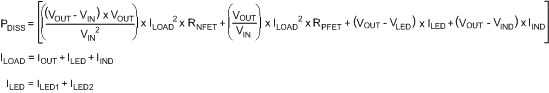Equation 11 consider the average current through the NFET and PFET. The actual power losses are higher due to the RMS currents and the quiescent power into IN. These, however, can give a decent approximation.

#### 7.2.2.7.2 LED Boost Mode

In LED mode with VOUT > VIN the device boost converter switches and make VOUT = VLED + 0.3 V. In this situation the total power dissipated in the LM3554 is approximated as:

Equation 12.#### 7.2.2.7.3 LED Pass Mode

In LED mode with VIN – ILOAD × RPFET > VLED + 0.3 V, the LM3554 operates in pass mode. In this case, the NFET is off, and the PFET is fully on. The difference between VIN - ILOAD × RPMOS and VLED are dropped across the current sources. In this situation the total power dissipated in the LM3554 is approximated as:

Equation 13.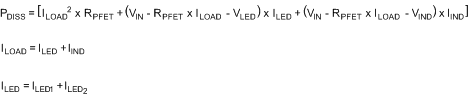Once the total power dissipated in the LM3554 is calculated the ambient temperature and the thermal resistance of the 16-pin DSBGA (YFQ package) are used to calculate the total die temperature (or junction temperature TJ).

As an example, assume the LM3554 is operating at VIN = 3.6 V and configured for voltage-output mode with VOUT = 5 V and IOUT = 0.7 A. The LED currents are then programmed in torch mode with 150 mA each at VLED = 3.6 V. Additionally, the indicator LED has 10 mA at VIND = 3.6 V. Using Equation 12 and Equation 13 above, the approximate total power dissipated in the device is:

Equation 14.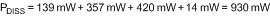The die temperature approximation is:

Equation 15.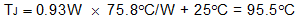In this case the device can operate at these conditions. If then the ambient temperature is increased to 85°C, the die temperature would be 140.8°C; thus, the die temperature would be above the absolute maximum ratings, and the load current would need to be scaled back. This example demonstrates the steps required to estimate the amount of current derating based upon operating mode, circuit parameters, and the device's junction-to-ambient thermal resistance. In this example a thermal resistance of 75.8°C/W was used (JESD51-7 standard). Because thermal resistance from junction-to-ambient is largely PCB layout dependent, the actual number used likely may be different and must be taken into account when performing these calculations.

#### 7.2.3 Application Curves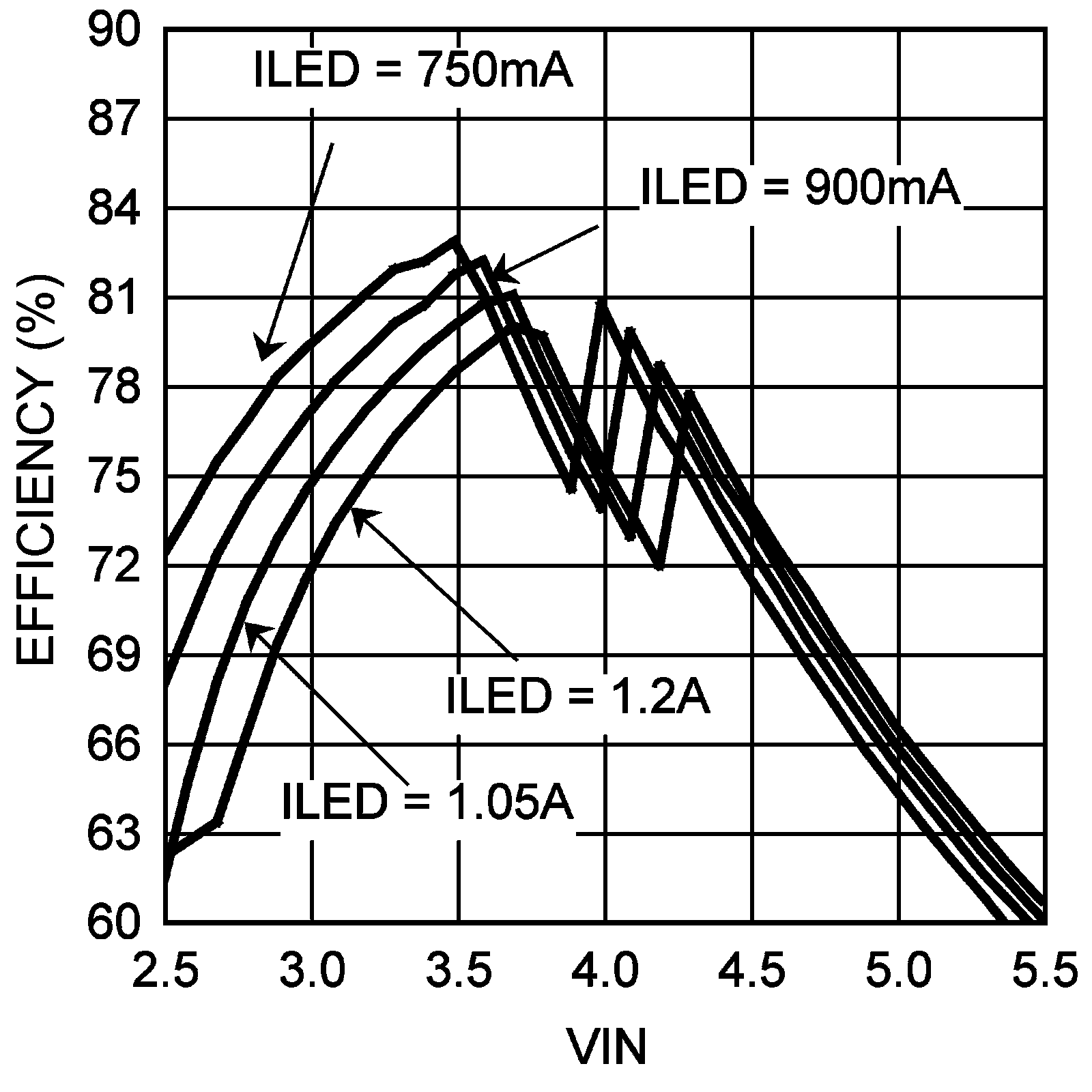Single LED
Figure 45. LED Efficiency vs VIN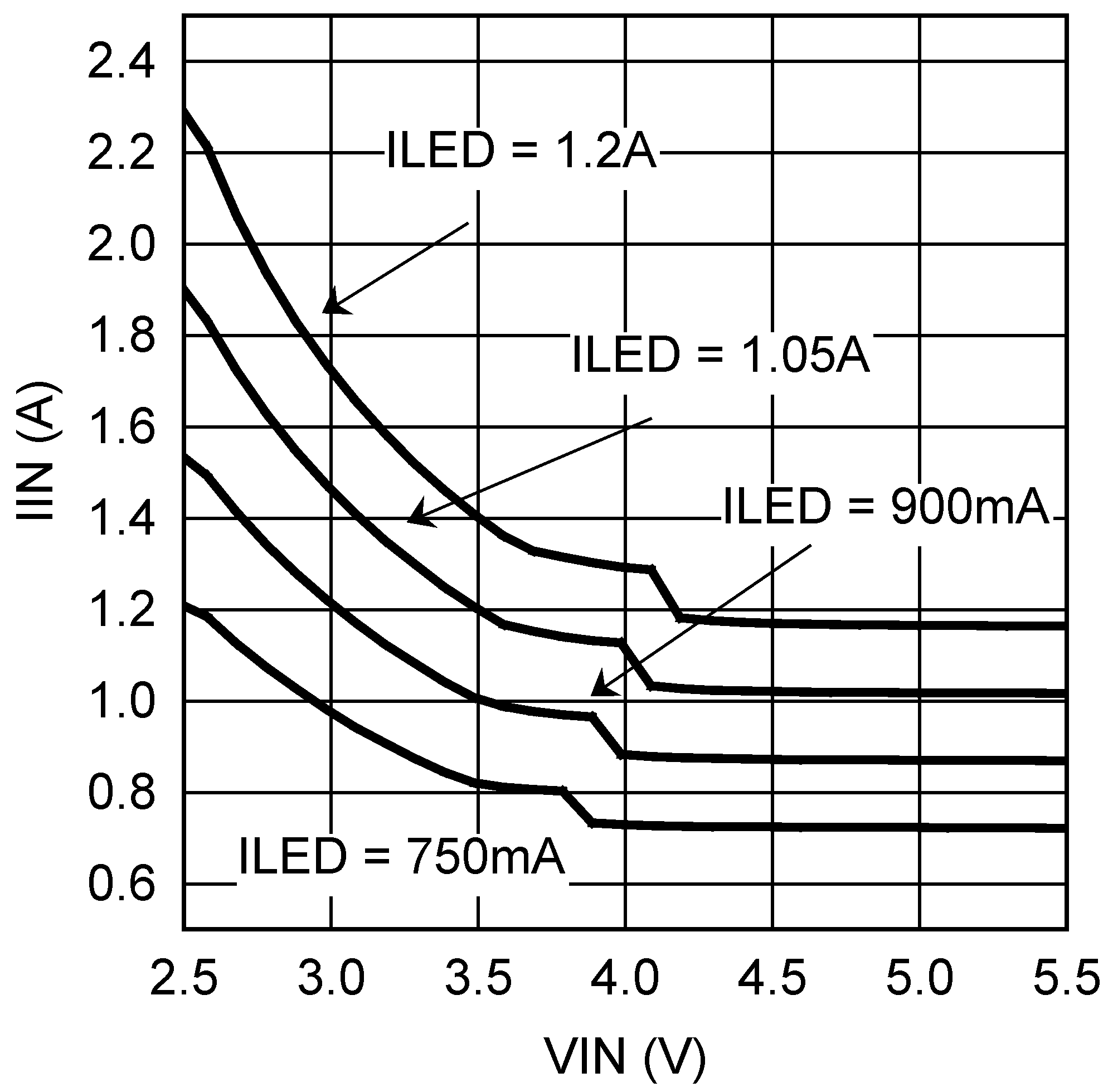Single LED
Figure 47. Input Current vs VIN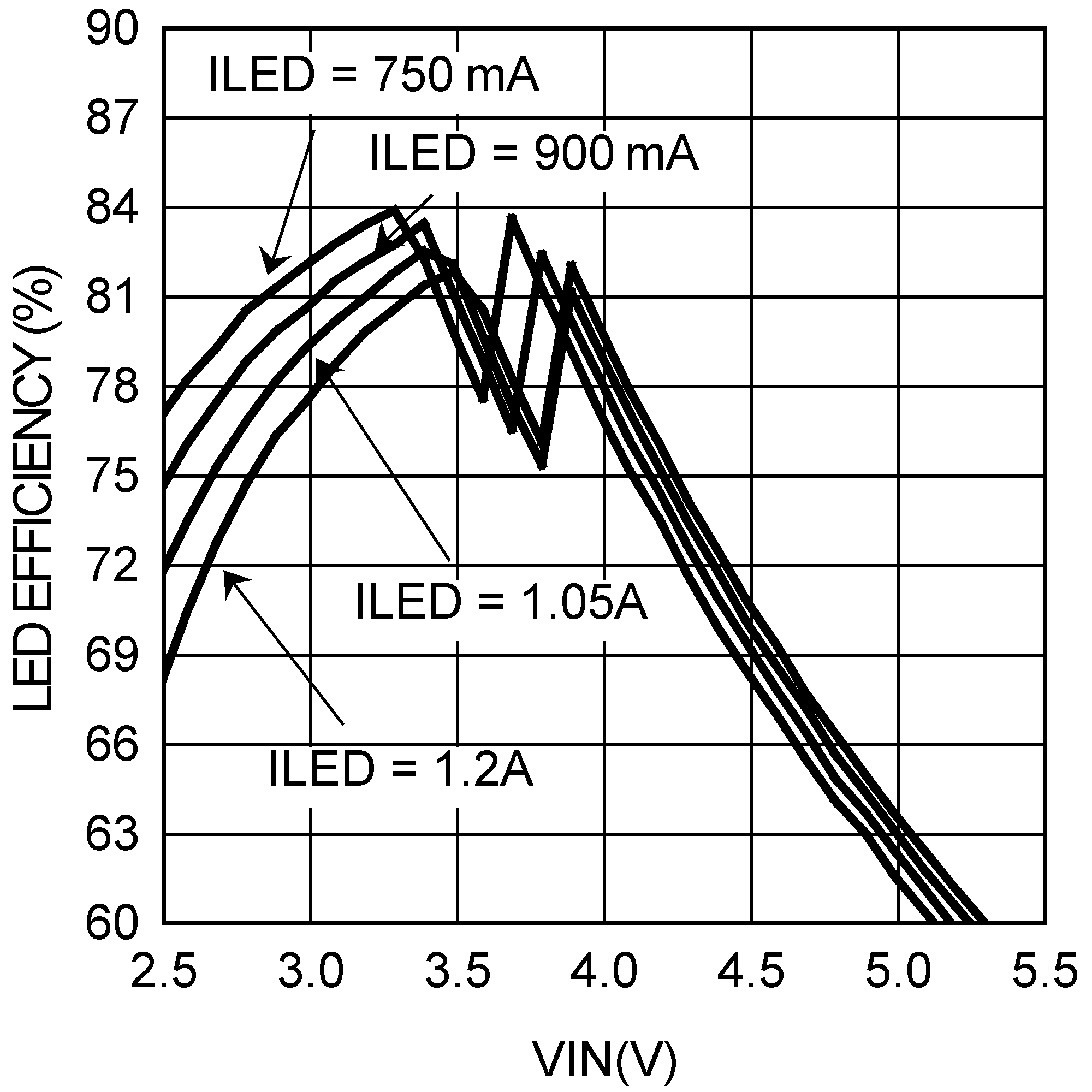Dual LEDs L = Coilcraft LPS4018-222
Figure 49. LED Efficiency vs VINVOUT = 5 V Voltage-Output Mode
Figure 51. Efficiency vs IOUT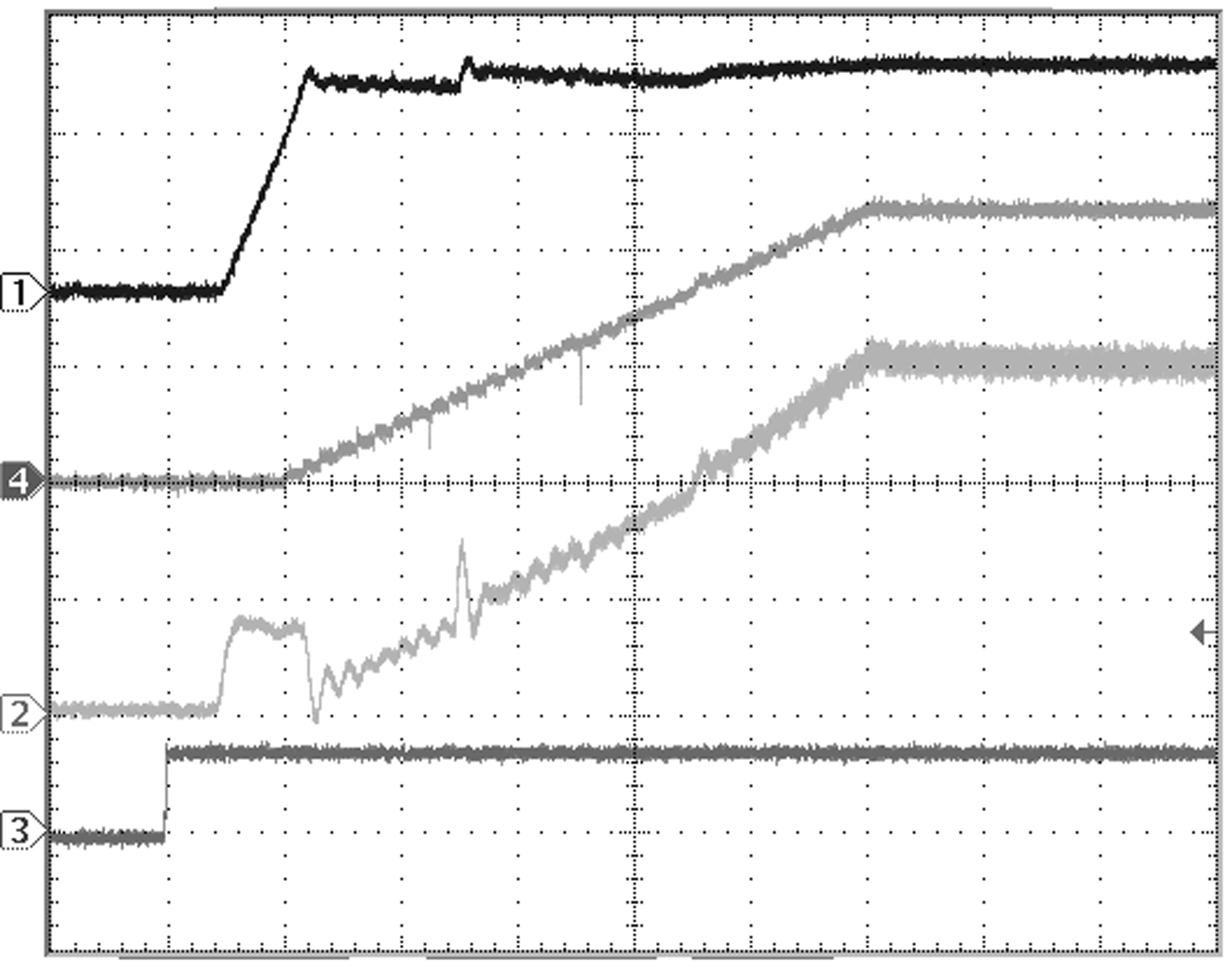Time Base: 100 µs/div IFLASH = 1.2 A Single LED Ch 1: VOUT (2 V/div) Ch 2: IL (500 mA/div) Ch 4: ILED (500 mA/div) Ch 3: STROBE (5 V/div)
Figure 53. Start-Up Into Flash Mode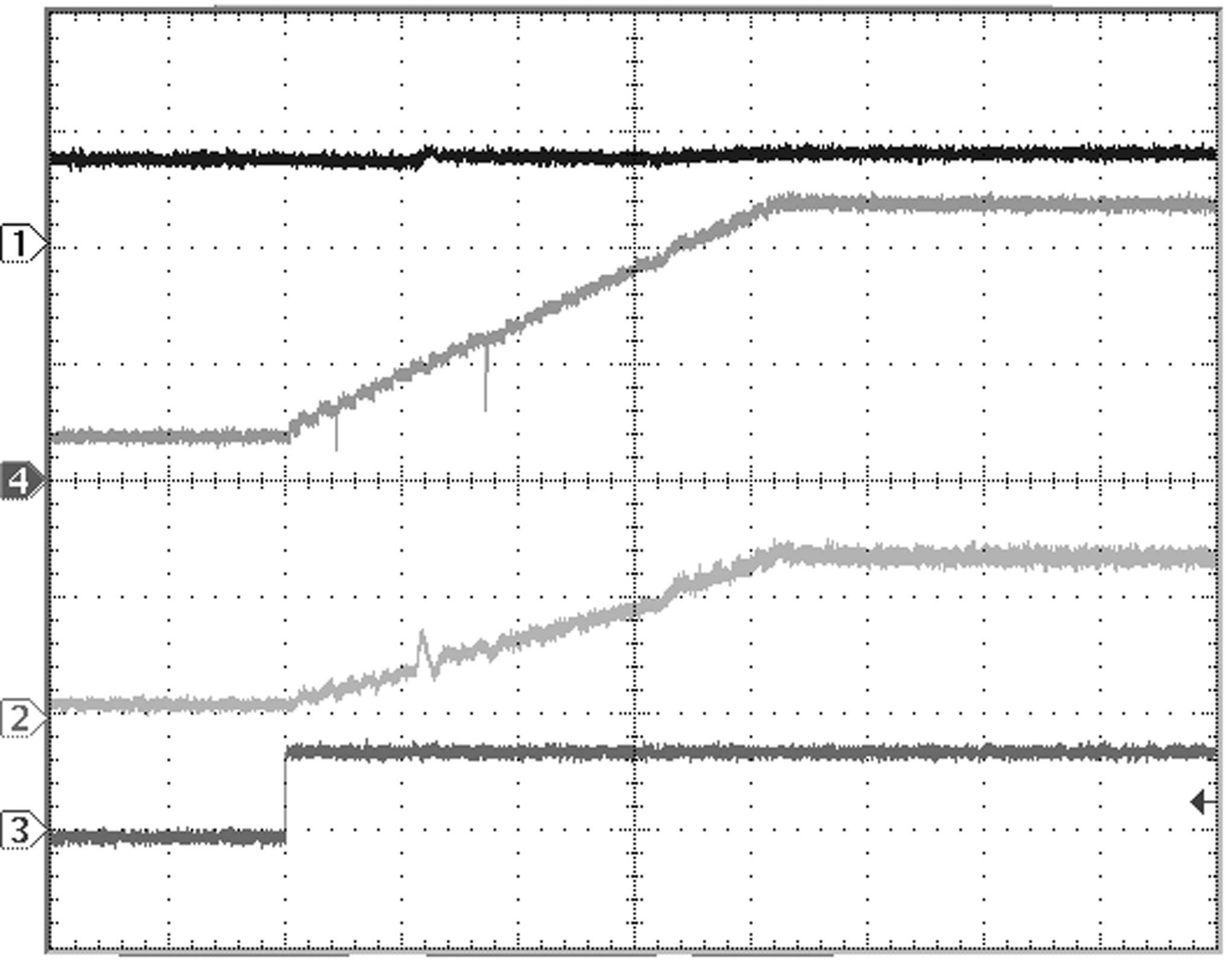Time Base: 100 µs/div IFLASH = 1.2 A ITORCH = 295 mA Ch 1: VOUT (5 V/div) Ch 2: IL (1 A/div) Single LED Ch 4: ILED (500 mA/div) Ch 3: STROBE (5 V/div)
Figure 55. Torch Mode to Flash Mode Transition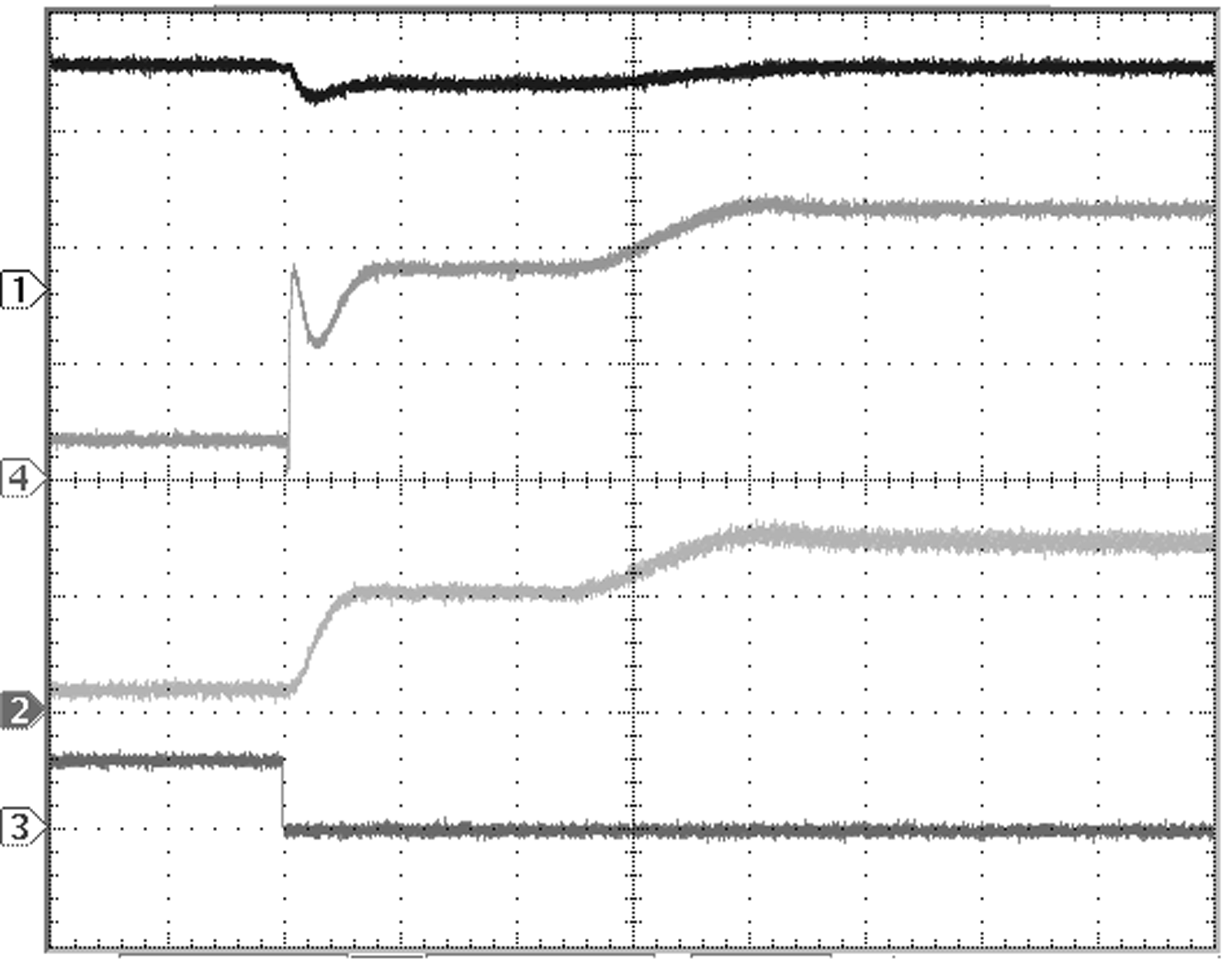Time Base: 20 µs/div IFLASH = 1.2 A ITORCH = 180 mA Ch 1: VOUT (2 V/div) Ch 2: IL (1 A/div) Single LED Ch 4: ILED (500 mA/div) Ch 3: TX1 (5 V/div)
Figure 57. TX1 Interrupt Operation, TX1 Falling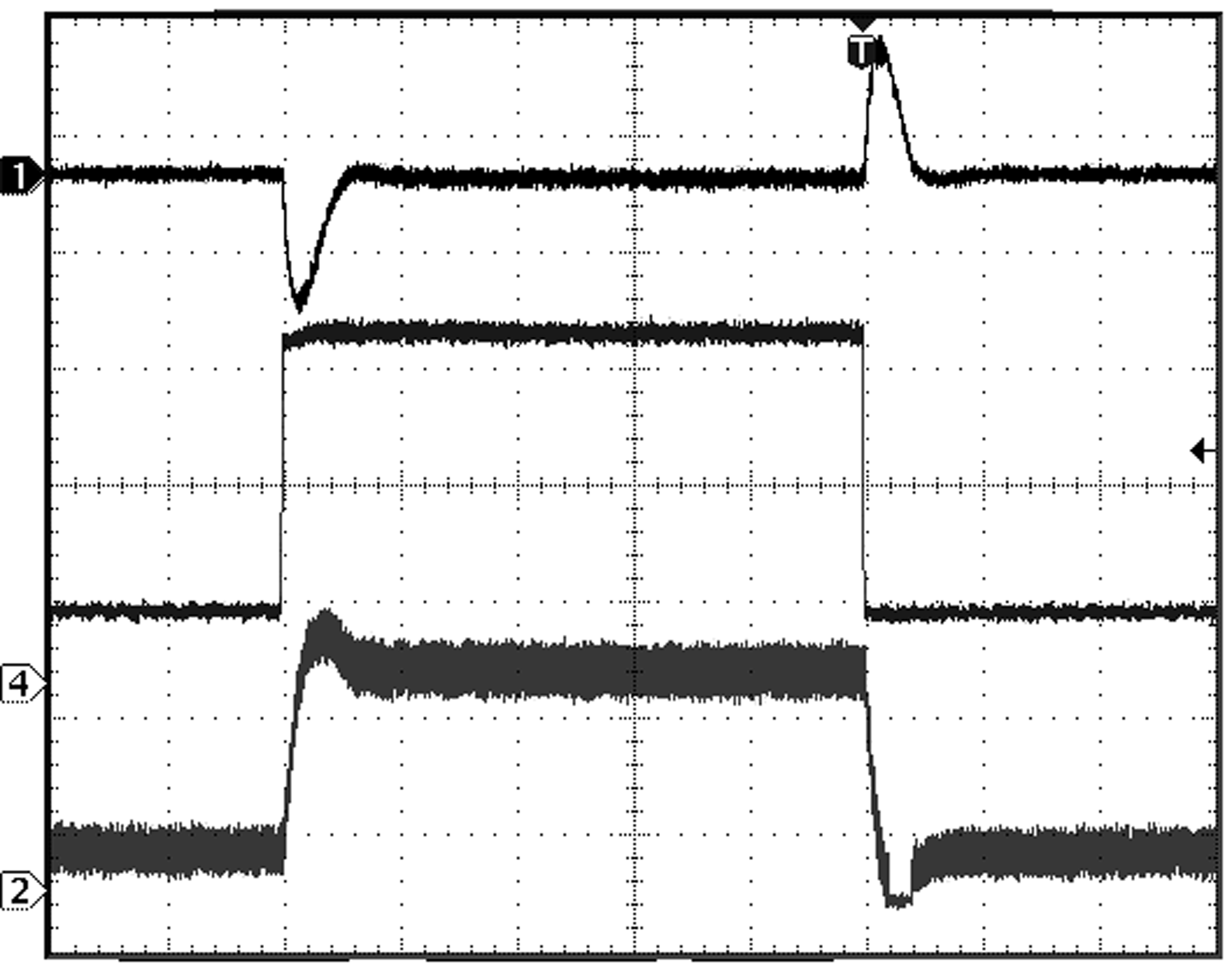Time Base: 40 µs/div VIN = 3.6 V VOUT = 5 V Ch 1: VOUT (500 mV/div) Ch 2: IL (500 mA/div) Ch 4: IOUT (500 mA/div)
Figure 59. Load Transient (Voltage Output Mode)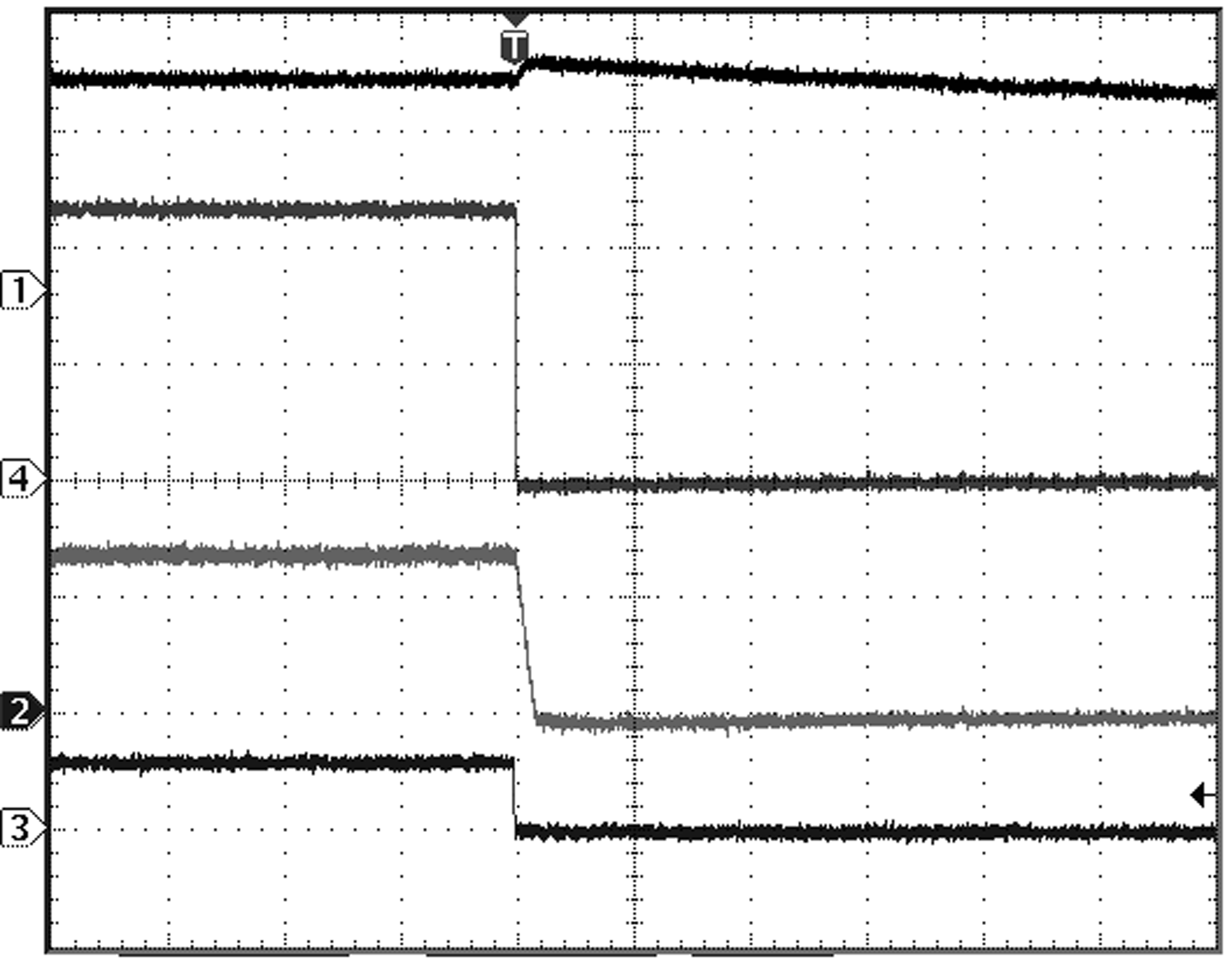Time Base: 20 µs/div ILED = 1.2 A Single LED Ch 1: VOUT (2 V/div) Ch 3: HWEN (5 V/div) Ch 4: ILED (500 mA/div)
Figure 61. Flash Pulse to HWEN Low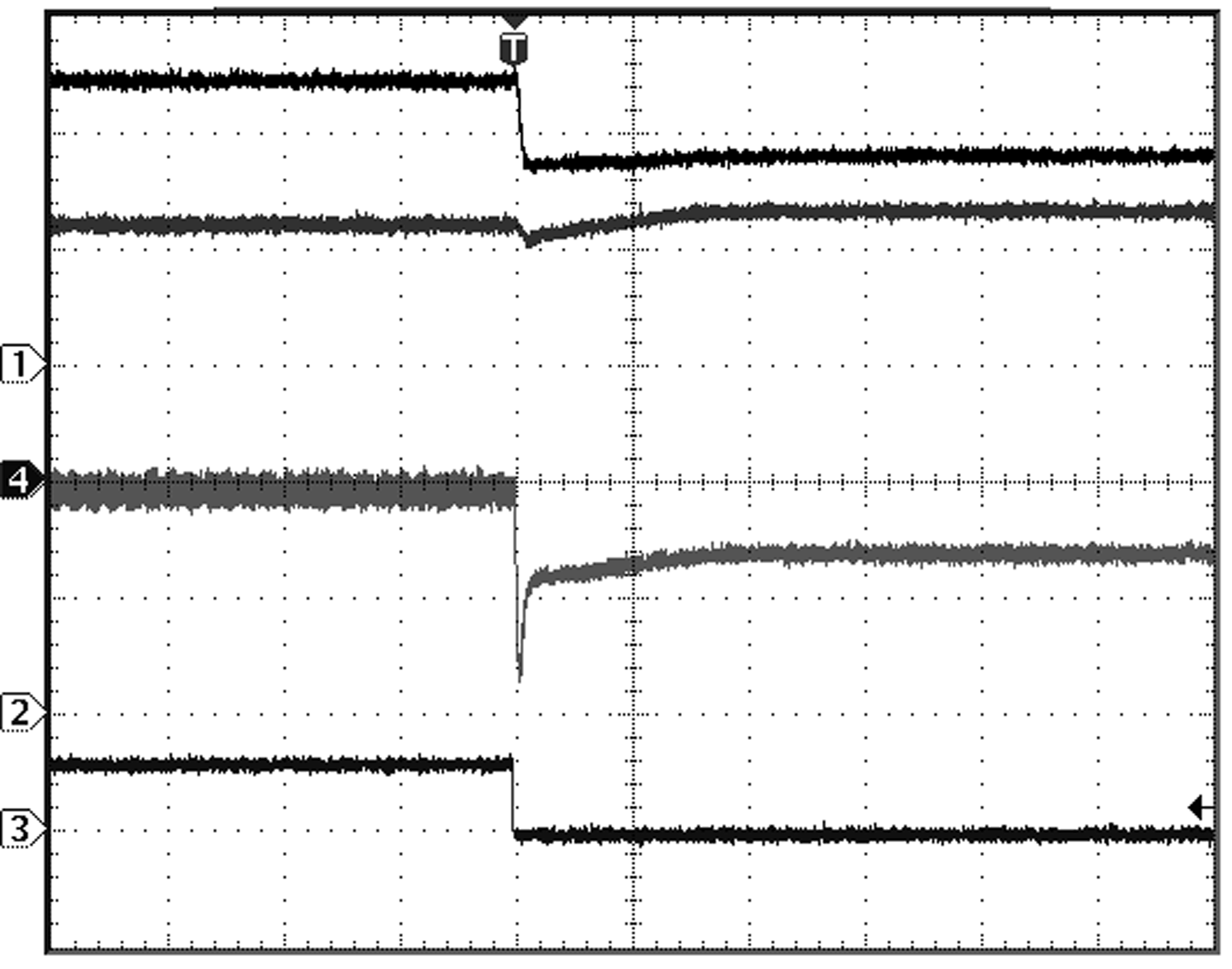Time Base: 100 µs/div ILED = 1.2 A VOUT = 5 V Ch 1: VOUT (2 V/div) Ch 2: IL (1 A/div) Single LED Ch 4: ILED (500mA/div) Ch 3: ENVM (5 V/div)
Figure 63. Flash Pulse and VOUT to Flash PulseTime Base: 100 ms/div ILED = 1.2 A 3.1-V UVLO Setting Ch 3: VIN (1V/div) Ch 4: ILED (500 mA/div) Single LED
Figure 65. VIN Monitor Response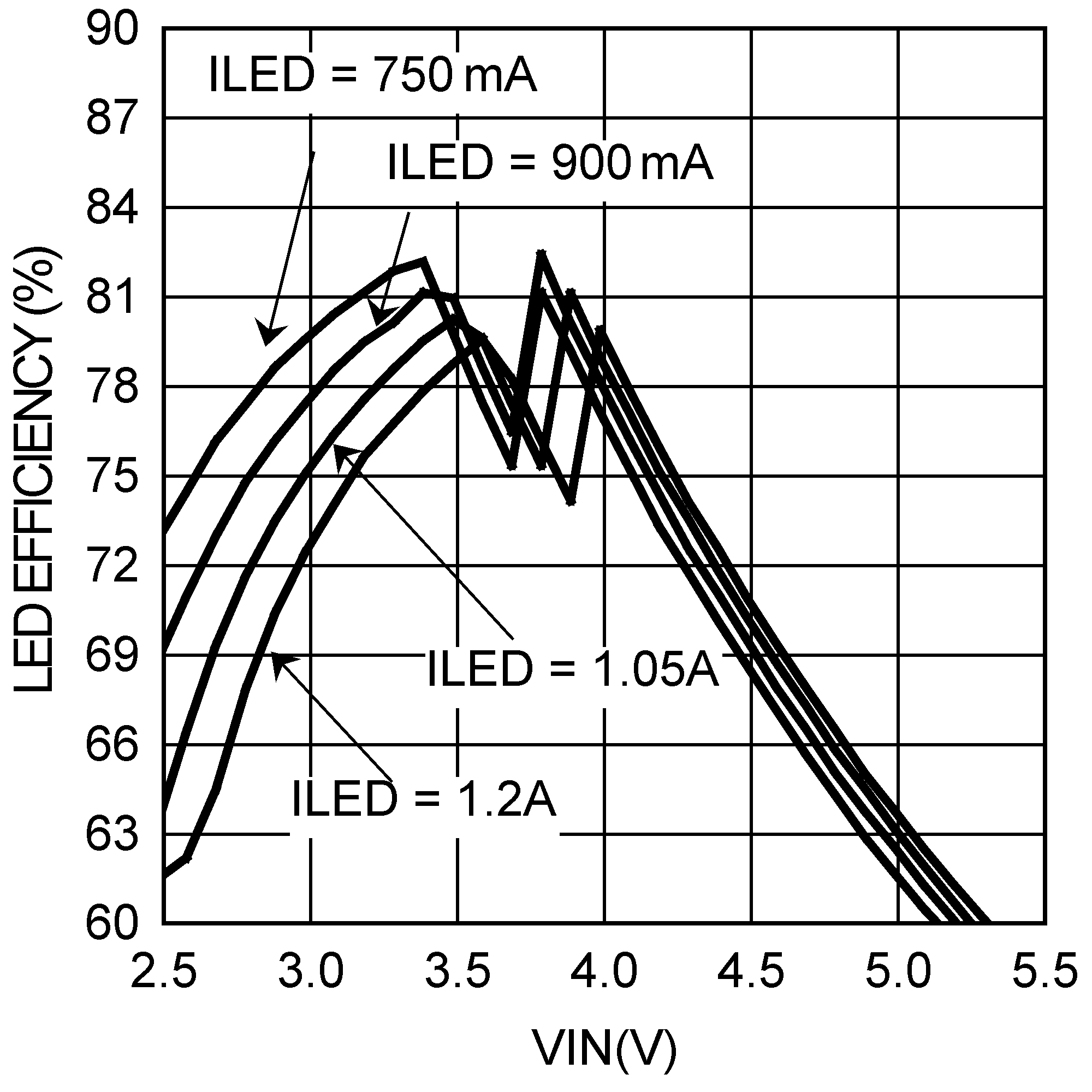Dual LEDs
Figure 46. LED Efficiency vs VIN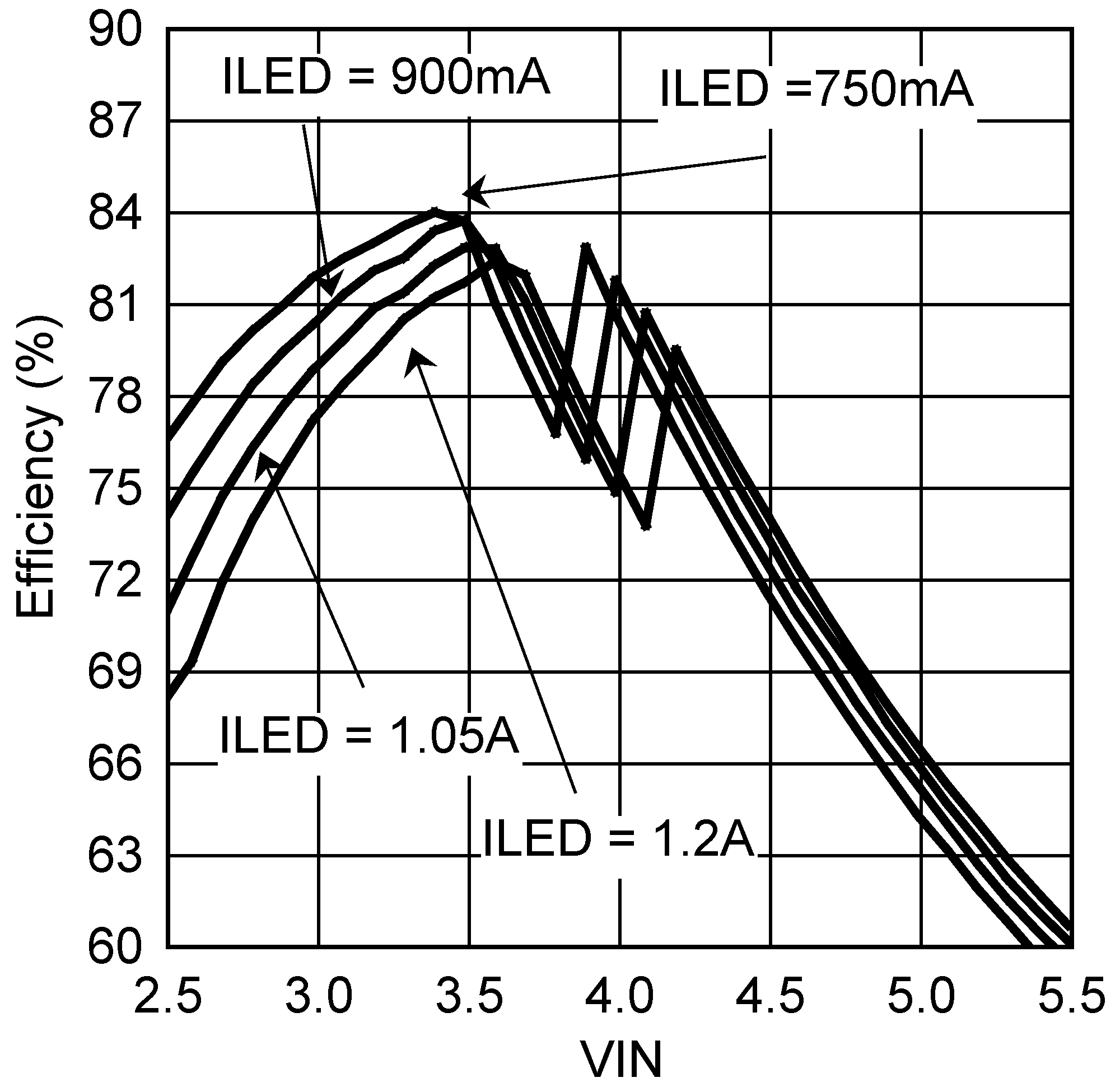Single LED L = Coilcraft LPS4018-222
Figure 48. LED Efficiency vs VINSingle LED L = Coilcraft LPS4018-222
Figure 50. Input Current vs VIN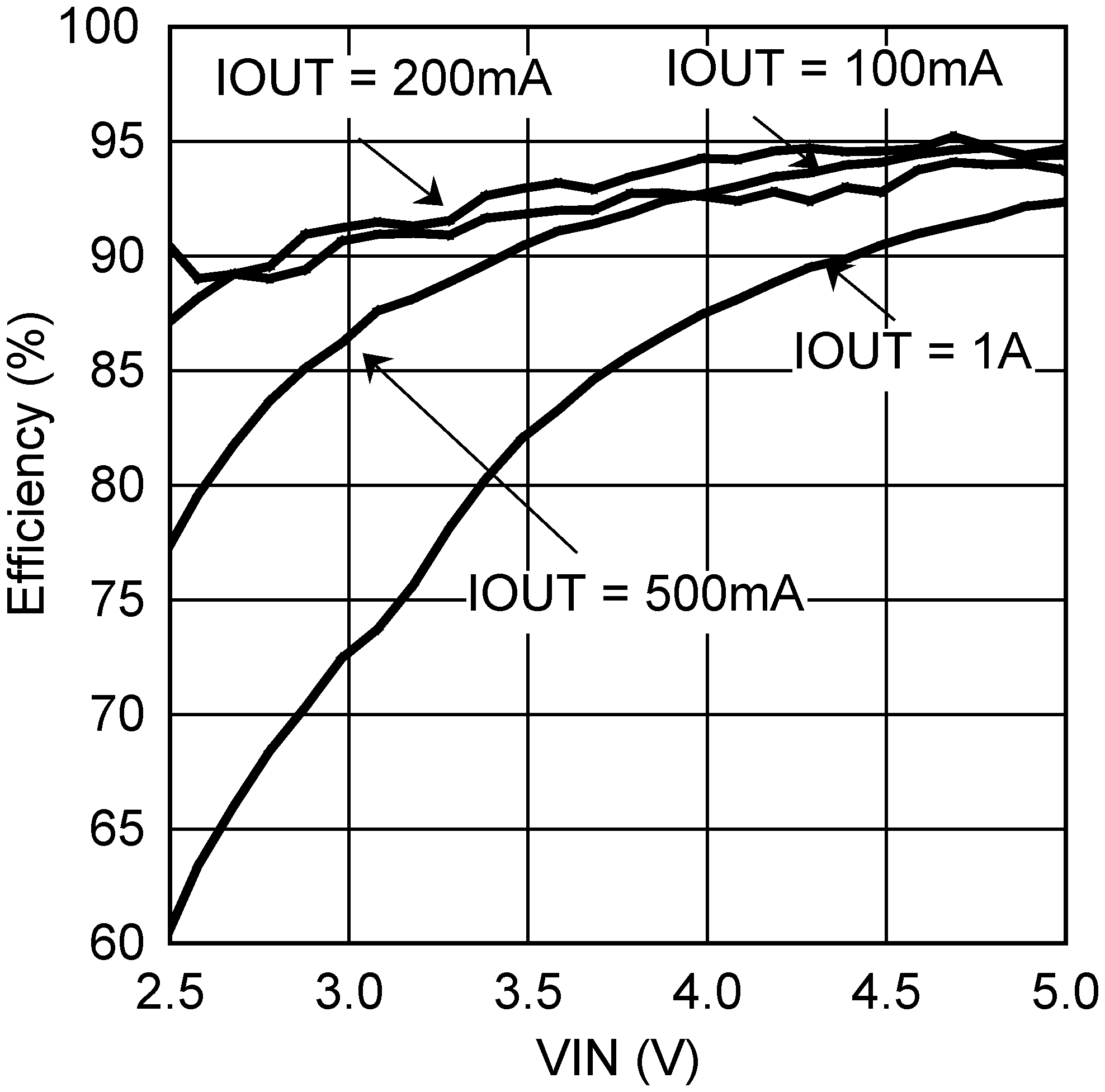VOUT = 5 V Voltage-Output Mode
Figure 52. Efficiency vs VINTime Base: 100 µs/div ITORCH = 180 mA 90-mA Torch Setting Single LED Chl 1: VOUT (2 V/div) Ch 2: IL (500 mA/div) Ch 4: ILED (100 mA/div) Ch 3: TX1 (5 V/div)
Figure 54. Start-Up Into Hardware Torch Mode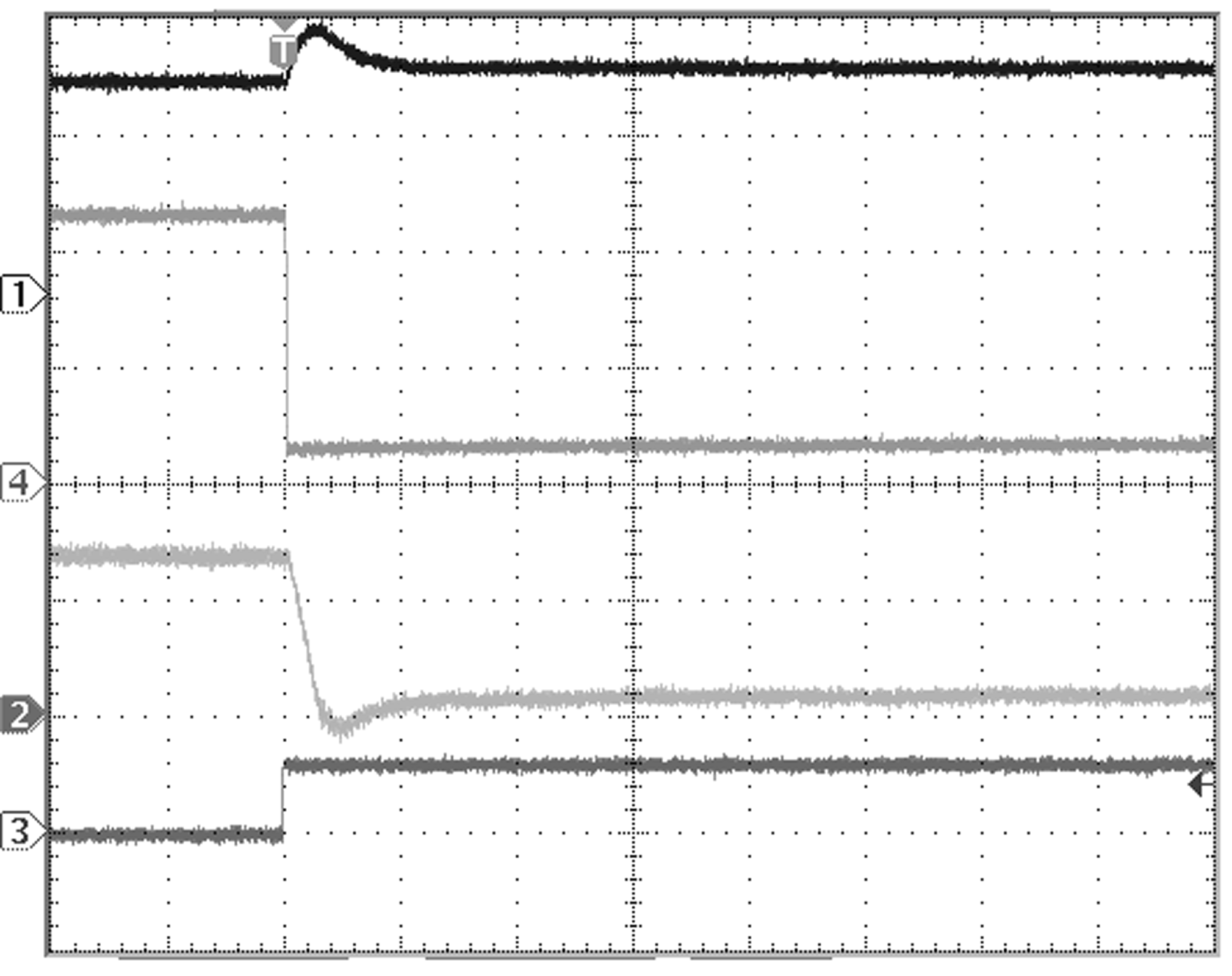Time Base: 20 µs/div IFLASH = 1.2 A ITORCH = 180 mA Ch 1: VOUT (2 V/div) Ch 2: IL (1 A/div) Single LED Ch 4: ILED (500 mA/div) Ch 3: TX1 (5 V/div)
Figure 56. TX1 Interrupt Operation, TX1 Rising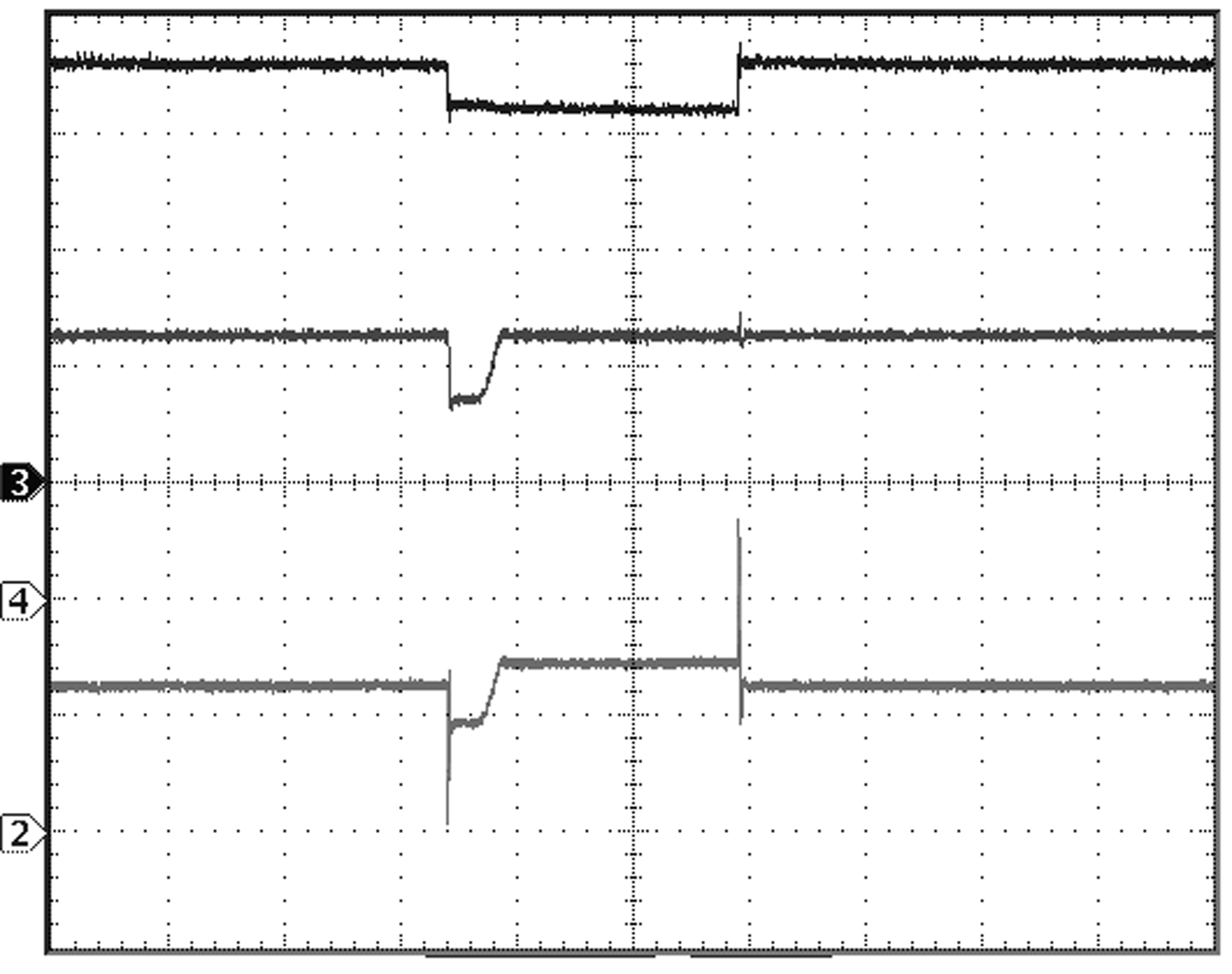Time Base: 400 µs/div IFLASH = 1.2 A Single LED Ch 3: VIN (5 V/div) Ch 2: IL (1 A/div) Ch 4: ILED (500 mA/div)
Figure 58. Line Transient (LED Mode)Time Base: 200 µs/div VOUT = 5 V IOUT = 500 mA Ch 1: VOUT = (5 V/div) Ch 2: IL + IIN (500 mA/div) Ch 3 (Top Trace): VIN (1 V/div)
Figure 60. Line Transient (Voltage Output Mode)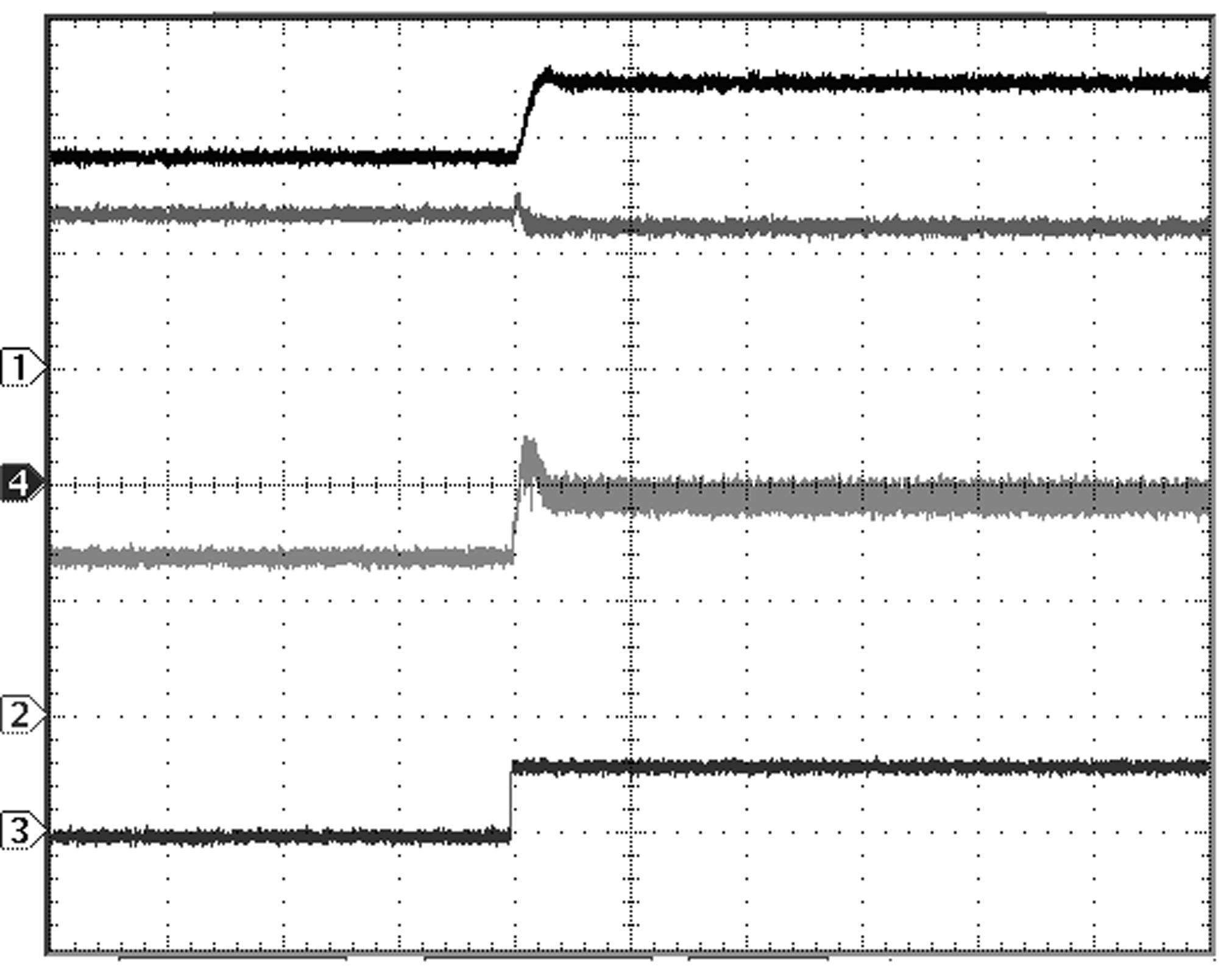Time Base: 100 µs/div VOUT = 5 V ILED = 1.2 A Ch 1: VOUT (2 V/div) Ch 2: IL (1 A/div) Single LED Ch 4: ILED (500 mA/div) Ch 3: ENVM (5 V/div)
Figure 62. Flash Pulse to Flash Pulse + VOUT Mode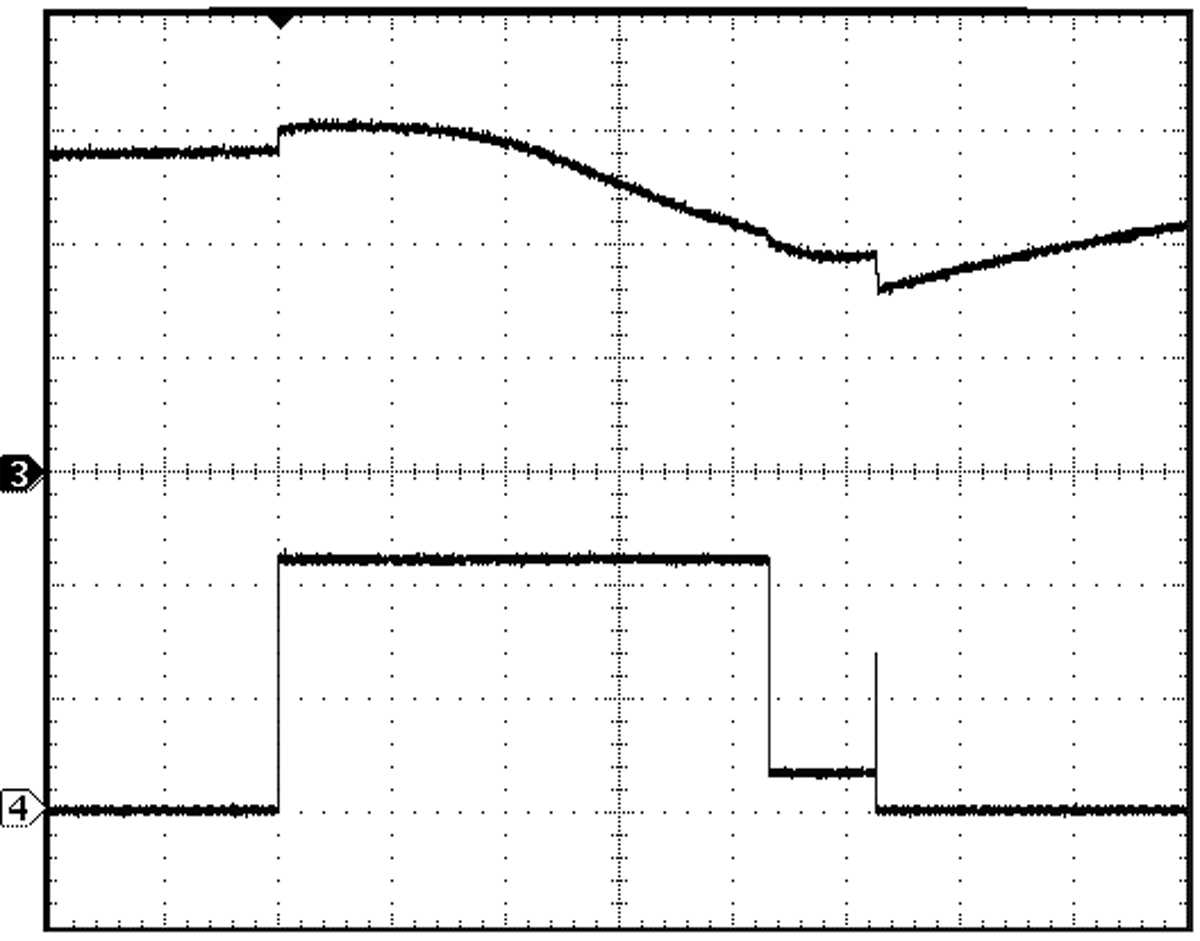Time Base: 200 µs/div Circuit of Figure 43 Single LED ILED = 1.2 A Ch 3: NTC pin voltage (5 V/div) Ch 4: ILED (500 mA/div) R(T) = 100 kΩ at 25°C R3 = 9 kΩ
Figure 64. NTC Mode Response